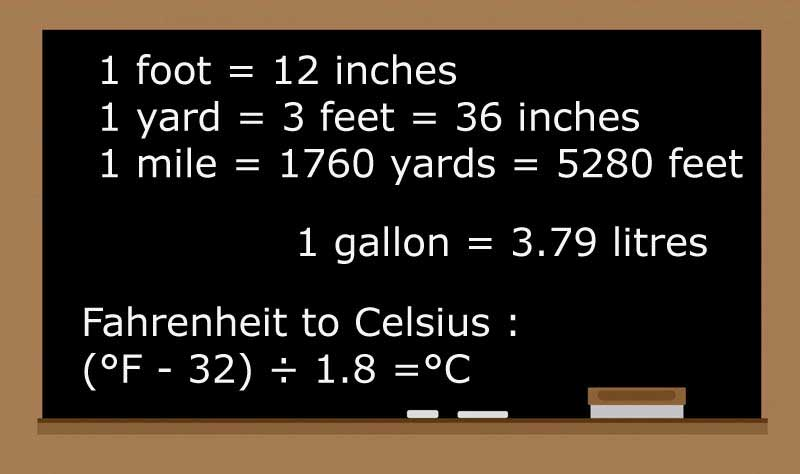## Units of Measurement

LENGTH
Length (GB, USA) - inch (in), foot (ft), yard (yd), mile

1 foot = 12 inches
1 yard = 3 feet = 36 inches
1 mile = 1760 yards = 5280 feet

Length (metric) - millimeter (mm), centimeter (cm), decimeter (dm), meter (m), kilometer (km)

1 cm = 10 mm
1 dm = 10 cm
1 dm = 100 mm
1 m = 1000 mm
1 m = 100 cm
1 m = 10dm
1 km = 1,000,000 mm
1 km = 100,000 cm
1 km = 10,000 dm
1 km = 1000 m

Converting Between Systems

1 meter = 1.09 yards
1 meter= 39.37 inches
1 inch = 2.54 cm
1 foot = 30.48 cm
1 yard = 0.914 metres
1 mile = 1.61 km

AREA
Calculation of the area of a square whose length and width are 1 metre would be:
1 metre x 1 metre = 1 m²
Calculation of the area of a square whose length is 3 metres and width is 2 metres would be:
3 metres x 2 metres = 6 m²

1 foot = 12 inches
1 square foot = 144 sq inches
1 square yard = 9 sq feet
1 square mile = 3,097,600 sq yards
1 square mile = 27,878,400 sq feet

1 square kilometre = 1,000,000 sq metres
1 m²= 10,000 sq cm
1 cm² = 100 sq mm
100 m² = 1 are (a)
100 ares = 1 hectare (ha)
100 hectares = 1 km²

Converting Between Systems

1 square inch = 6.4516 cm²
1 square foot = 0.09290304 m²
1 square yard = 0.83612736 m²
1 square mile = 2.589988110336 km²

WEIGHT, MASS, VOLUME

Metric Volume
1 liter (l) = 1000 milliliters
1 liter (l) = 1000 cubic cm
1 hectoliter (hl) = 100 litres

US volume - US fluid ounces (fl oz), US cup (cp), US pint (pt), US quart (qt), and US gallon (gal)
1 US cup = 8 US fluid ounces
1 US pint = 2 US cups = 16 US fluid ounces
1 US quart = 2 US pints
1 US gallon = 4 US quarts = 8 US pints

Imperial volume - imperial fluid ounce (fl oz), imperial pint (pt), and imperial gallon (gal)
1 imperial pint = 20 imperial fluid ounces
1 imperial gallon = 8 imperial pints

Weight and mass are measured in ounces (oz) and pounds (lb), and stone (st) in imperial only
1 pound = 16 ounces
1 stone = 14 pounds

Metric weight
1 kilogram = 1,000 grams
1 metric ton = 1,000 kilograms
1 hectogram = 100 grams
1 dekagram = 10 grams
1 milligrams = 0.001 grams

Converting Between Systems

1 gallon = 3.79 litres
1 liter = 33.3 fluid ounces = 1.76 pints = 0.26 US gallons
1 ounce = 28.35 grams
1 pound = 0.45 kilograms
1 kilogram = 35.32 ounces = 2.2 pounds
1 metric ton = 32,000 oz
1 metric ton = 2,204.6 pounds

TEMPERATURE

Celsius- A scale and unit of measurement for temperature where 0 °C is the freezing point of water. Also known as centigrade.

Fahrenheit - A unit of measurement for temperature used most commonly in the United States.

Boiling water (at normal pressure) measures 100° in Celsius, but 212° in Fahrenheit.
And as water freezes it measures 0° in Celsius, but 32° in Fahrenheit

How to convert-
from Celsius to Fahrenheit: first multiply by 1.8, then add 32
from Fahrenheit to Celsius: first subtract 32, then multiply by 1.8

Celsius to Fahrenheit : (°C × 1.8) + 32 =°F
Fahrenheit to Celsius : (°F - 32) ÷ 1.8 =°C